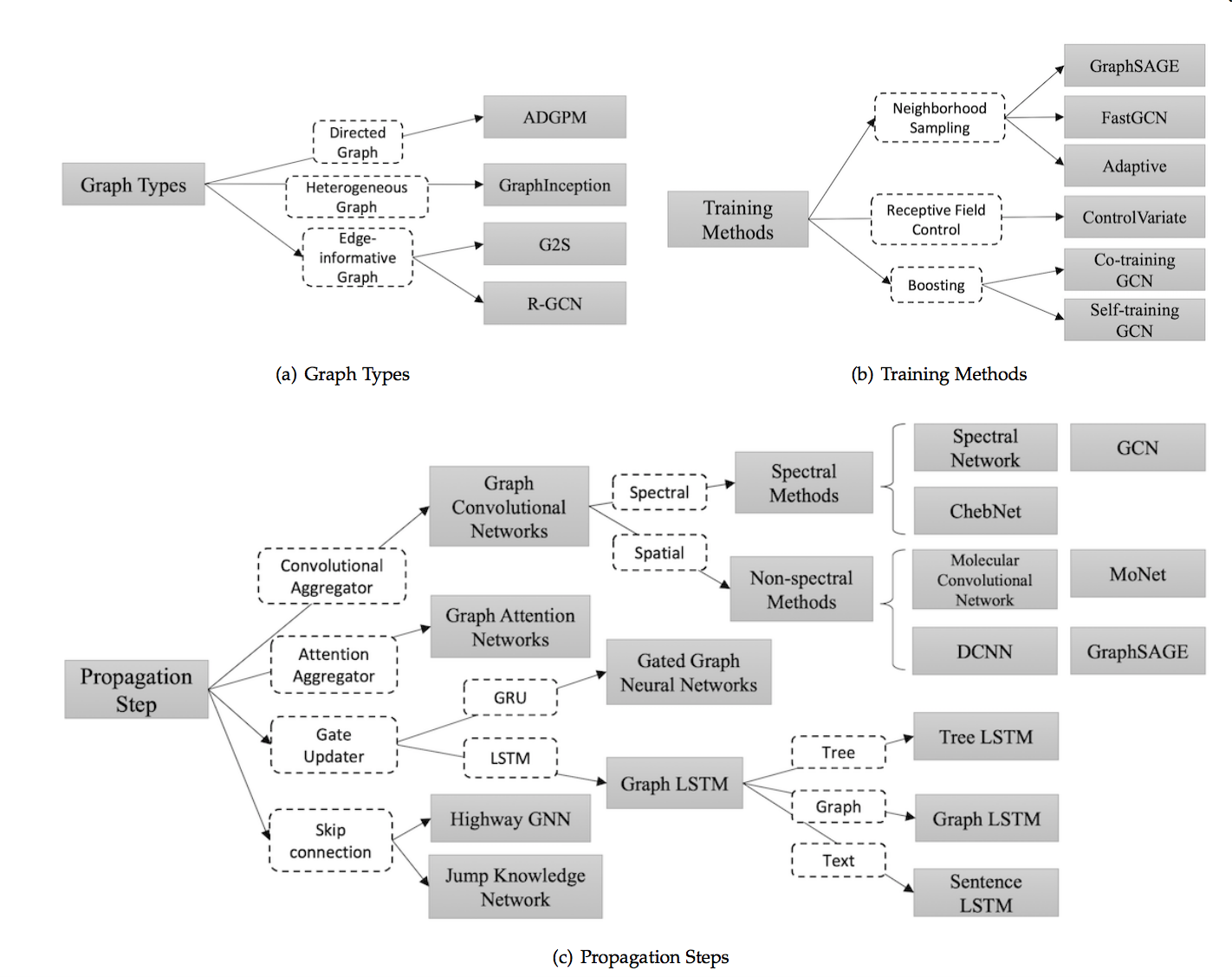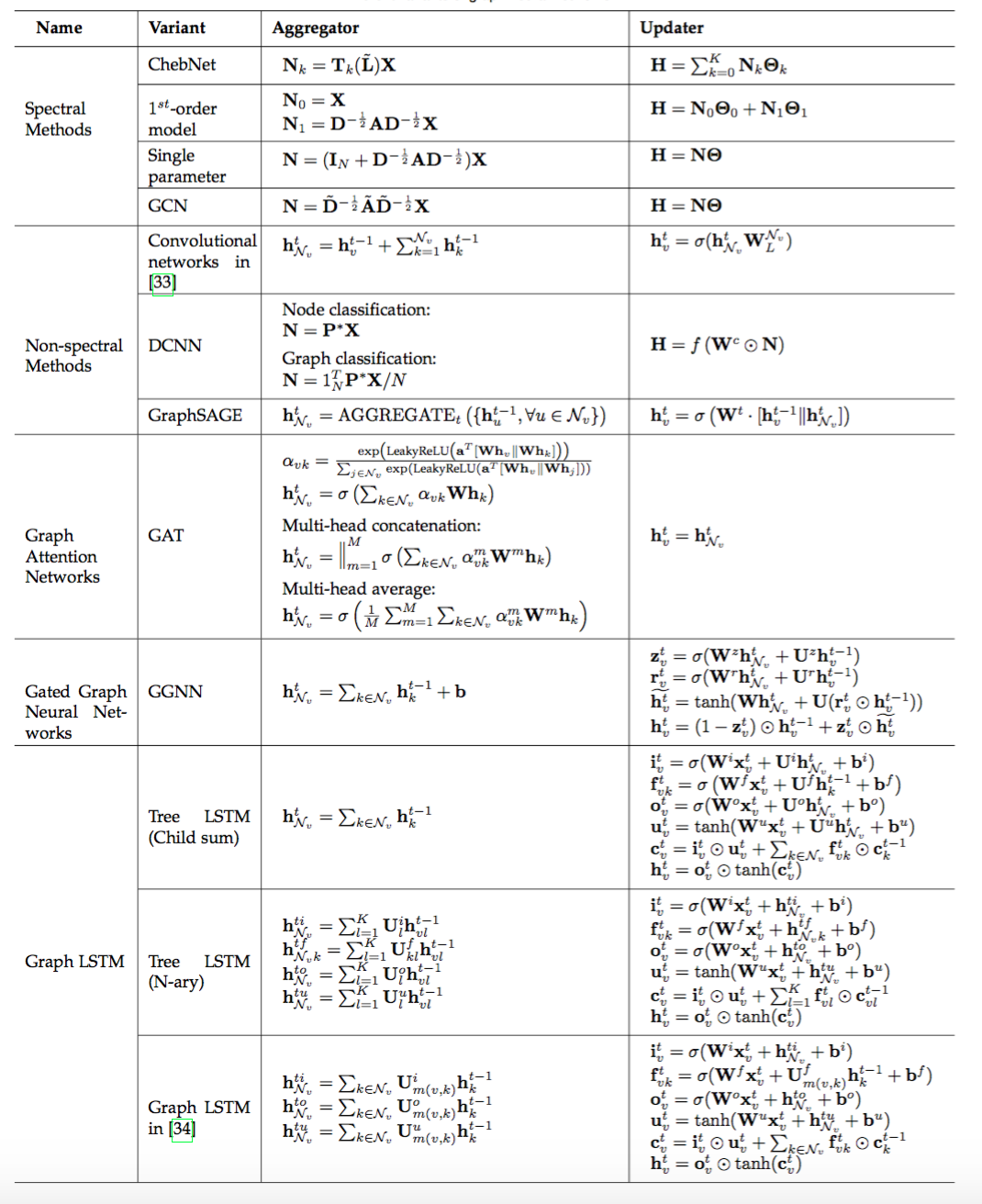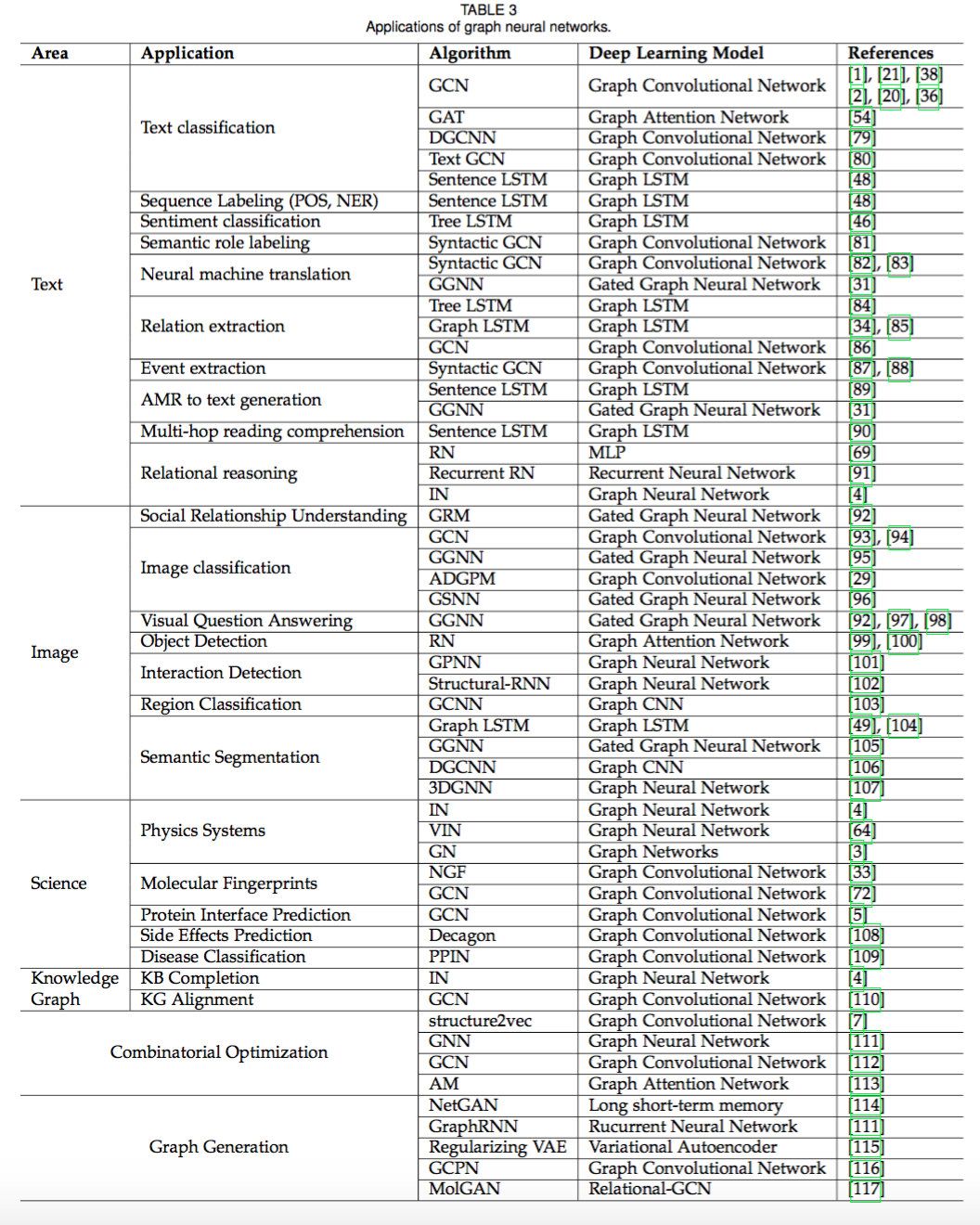To Top

## 概述

github链接：https://github.com/thunlp/GNNPapers

## 引言

GNN的第一个动机源于卷积神经网络（CNN）：CNN只能在规则的Euclidean数据上运行，如图像（2维网格）和文本（1维序列）。如何将CNN应用于图结构这一非欧几里德空间，成为GNN模型重点解决的问题。

GNN的另一个动机来自图嵌入（Graph Embedding），它学习图中节点子图的低维向量空间表示。DeepWalk、node2vec、LINE、SDNE等方法在网络表示学习领域取得了很大的成功。然而，这些方法在计算上较为复杂并且在大规模上的图上不是最优的，GNN旨在解决这些问题。

## 模型### 原始GNN

2009年的第一篇GNN的文章The graph neural network model。GNN的target是要学习包括了每个节点的邻居的信息的state embedding $$h_v\in R^s$$。这里的state embedding $$h_v$$是节点$$v$$的一个$$s$$维的向量，能用来产生输出$$o_v$$，例如节点的label。$$f$$是一个带有参数的函数，称为local transition function，被所有的节点共享，能根据输入的邻居来更新节点状态。$$g$$是local output function，表示如何产生output。所以：

$\begin{matrix} h_v=f(x_v,x_{co[v]},h_{ne[v]},x_{ne[v]})\\ o_v=g(h_v,x_v)\\ \end{matrix}$

• $$x_v$$：节点$$v$$的特征。
• $$x_{co[v]}$$：节点$$v$$的边的特征。
• $$h_{ne[v]}$$：节点$$v$$的邻居节点的state。
• $$x_{ne[v]}$$：节点$$v$$的邻居节点的特征。

$\begin{matrix} H=F(H,X)\\ O=G(H,X_N)\\ \end{matrix}$

$H^{t+1}=F(H^t,X)$

$loss=\sum ^p_{i=1}(t_i-o_i)$

### GNN变种

#### 图类型

##### Directed Graphs

$H^t=\sigma(D^{-1}_pA_p\sigma(D^{-1}_cA_cH^{t-1}W_c)W_p)$

#### 传播类型(propagation types)

propagation stepoutput step这两部是很重要的，主要分为以下类别：

• 卷积：Graph Convolutional Network（GCN）希望将卷积操作应用在图结构数据上，主要分为Spectral Method和Spatial Method（Non-spectral Method）两类。
• Spectral Method：希望使用谱分解的方法，应用图的拉普拉斯矩阵分解进行节点的信息收集。
• Spatial Method直接使用图的拓扑结构，根据图的邻居信息进行信息收集。
• 注意力机制：Graph Attention Network 致力于将注意力机制应用在图中的信息收集阶段
• 门机制：门机制应用于节点更新阶段。Gated graph neural network 将GRU机制应用于节点更新。很多工作致力于将 LSTM应用于不同类型的图上，主要包括Tree LSTMGraph LSTMSentence LSTM
• 残差连接：堆叠多层图神经网络可能引起噪声在指数级增加的邻居中传播，所以很多工作将残差机制应用于图神经网络中。主要包括Highway GNNJump Knowledge Network两种不同的处理方式。### General Frameworks

#### Message Passing Neural Networks(MPNN)

MPNN将模型总结为信息传递阶段和节点更新阶段，概括了多种图神经网络和图卷积神经网络方法。

#### Non-local Neural Networks(NLNN)

NLNN总结了很多种基于自注意力机制的方法。

#### Graph Networks(GN)

GN提出了更加通用的模型，能够总结概括几乎所有文中提到的框架，并且拥有更加灵活的表示能力、易于配置的块内结构以及易于组合的多模块架构。

## 应用### 结构化场景

GNN 被广泛应用在社交网络、推荐系统、物理系统、化学分子预测、知识图谱等领域。

## 开放性问题

1. 浅层结构。经验上使用更多参数的神经网络能够得到更好的实验效果，然而堆叠多层的GNN却会产生over-smoothing的问题。具体来说，堆叠层数越多，节点考虑的邻居个数也会越多，导致最终所有节点的表示趋向于一致
2. 动态图。目前大部分方法关注于在静态图上的处理，对于如何处理节点信息边信息随着时间步动态变化的图仍是一个开放问题。
3. 非结构化场景。虽然很多工作应用于非结构化的场景（比如文本），然而并没有通用的方法用于处理非结构化的数据。
4. 扩展性。虽然已经有一些方法尝试解决这个问题，将图神经网络的方法应用于大规模数据上仍然是一个开放性问题。

comment here..